Do you like the article?
Share it with others —
Use new possibilities of MetaTrader 5

#### Similar articles# MQL5: Create Your Own Indicator

MetaTrader 5Examples | 6 January 2010, 16:1263 5396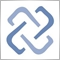### Introduction

What is an indicator? It is a set of calculated values that we want to be displayed on the screen in a convenient way. Sets of values are represented in programs as arrays. Thus, creation of an indicator means writing an algorithm that handles some arrays (price arrays) and records results of handling to other arrays (indicator values).

Despite the fact that there are a lot of ready indicators, which have become classics already, the necessity to create one's own indicators will always exist. Such indicators that we create using our own algorithms are called custom indicators. In this article we will discuss how to create a simple custom indicator.

### Indicators Are Different

An indicator can be presented as colored lines or areas, or it can be displayed as special labels pointing at favorable moments for position entering. Also these types can be combined, which gives even more indicator types. We'll consider creation of an indicator on the example of the well-known True Strength Index developed by William Blau.

### True Strength Index

The TSI indicator is based on the double-smoothed momentum to identify trends, as well as oversold/overbought areas. Mathematical explanation of it can be found in Momentum, Direction, and Divergence by William Blau. Here we include only its calculation formula.

TSI(CLOSE,r,s) =100*EMA(EMA(mtm,r),s) / EMA(EMA(|mtm|,r),s)

where:

• mtm = CLOSEcurrent – CLOSprev, array of values denoting the difference between close prices of the current bar and that of the previous one;
• EMA(mtm,r) = exponential smoothing of mtm values with the period length equal to r;
• EMA(EMA(mtm,r),s) = exponential smoothing of EMA(mtm,r) values with s period;
• |mtm| = absolute values mtm;
• r = 25,
• s = 13.

From this formula, we can extract three parameters that influence the indicator calculation. These are periods r and s, as well as the type of prices used for calculations. In our case we use CLOSE price.

### MQL5 Wizard

Let's display TSI as a blue line - here we need to start MQL5 Wizard. At the first stage we should indicated the type of a program we want to create - custom indicator. At the second stage, let's set the program name, r and s parameters and their values.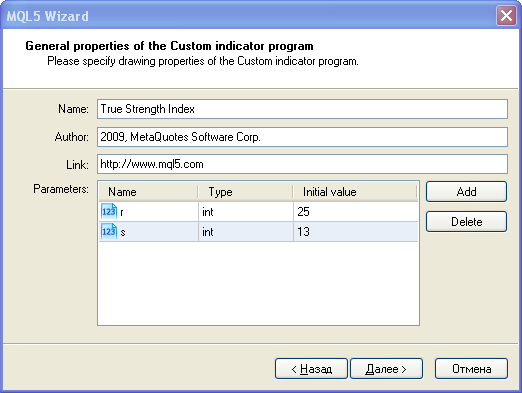After that let's define that the indicator shall be displayed in a separate window as a blue line and set the TSI label for this line.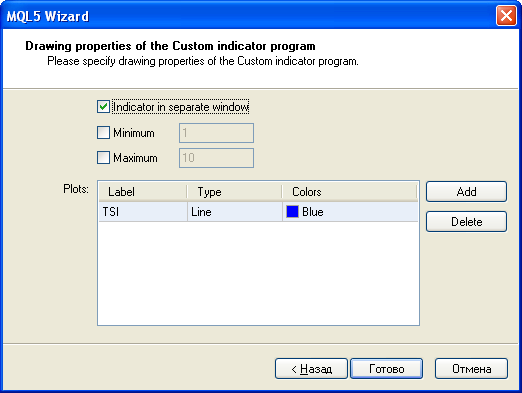All initial data have been entered, so we press Done and get a draft of our indicator.

```//+------------------------------------------------------------------+
//|                                          True Strength Index.mq5 |
//|                        Copyright 2009, MetaQuotes Software Corp. |
//|                                              https://www.mql5.com |
//+------------------------------------------------------------------+
#property copyright "2009, MetaQuotes Software Corp."
#property version   "1.00"
#property indicator_separate_window
#property indicator_buffers 1
#property indicator_plots   1
//---- plot TSI
#property indicator_label1  "TSI"
#property indicator_type1   DRAW_LINE
#property indicator_color1  Blue
#property indicator_style1  STYLE_SOLID
#property indicator_width1  1
//--- input parameters
input int      r=25;
input int      s=13;
//--- indicator buffers
double         TSIBuffer[];
//+------------------------------------------------------------------+
//| Custom indicator initialization function                         |
//+------------------------------------------------------------------+
int OnInit()
{
//--- indicator buffers mapping
SetIndexBuffer(0,TSIBuffer,INDICATOR_DATA);
//---
return(0);
}
//+------------------------------------------------------------------+
//| Custom indicator iteration function                              |
//+------------------------------------------------------------------+
int OnCalculate(const int rates_total,
const int prev_calculated,
const datetime& time[],
const double& open[],
const double& high[],
const double& low[],
const double& close[],
const long& tick_volume[],
const long& volume[],
{
//---
//--- return value of prev_calculated for next call
return(rates_total);
}
//+------------------------------------------------------------------+```

MQL5 Wizard creates the indicator header, in which it writes indicator properties, namely:

• indicator is displayed in a separate window;
• number of indicator buffers, indicator_buffers=1;
• number of plottings, indicator_plots= 1;
• name of plotting No 1, indicator_label1="TSI";
• style of the first plotting - line, indicator_type1=DRAW_LINE;
• color of plotting No 1, indicator_color1=Blue;
• style of a line, indicator_style1=STYLE_SOLID;
• line width for plotting 1, indicator_width1=1.

All preparations are ready, now we can refine and improve our code.

### OnCalculate()

The OnCalculate() function is the handler of the Calculate event, that appears when it's necessary to recalculate indicator values and redraw it on the chart. This is the event of a new tick receiving, symbol history update, etc. That's why the main code for all calculations of indicator values must be located exactly in this function.

Of course, auxiliary calculations can be implemented in other separate functions, but those functions must be used in the OnCalculate handler.

By default, MQL5 Wizard creates the second form of OnCalculate(), which provides access to all types of timeseries:

• Open, High, Low, Close prices;
• volumes (real and/or tick);
• period opening time.

But in our case we need only one data array, that's why let's change OnCalculate() the first form of calling.

```int OnCalculate (const int rates_total,      // size of the price[] array
const int prev_calculated,  // number of available bars at the previous call
const int begin,            // from what index in price[] authentic data start
const double& price[])      // array, on which the indicator will be calculated
{
//---
//--- return value of prev_calculated for next call
return(rates_total);
}  ```

This will enable us to further apply the indicator not only to price data, but also create the indicator based on values of other indicators.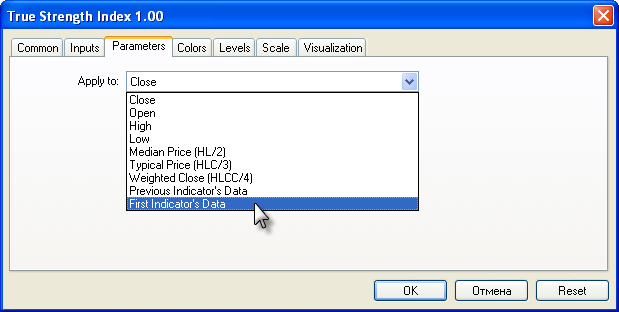If we select Close in the Parameters tab (it is offered by default), then price[] passed to OnCalculate() will contain close prices. If we select, for example, Typical Price, price[] will contain prices of (High+Low+Close)/3 for each period.

The rates_total parameter denotes the size of the price[] array; it will be useful for organizing calculations in a cycle. Indexing of elements in price[] starts from zero and is directed from past to future. I.e. the price element contains the oldest value, while price[rates_total-1] contains the latest array element.

### Organizing Auxiliary Indicator Buffers

Only one line will be shown in a chart, i.e. data of one indicator array. But before that we need to organize intermediate calculations. Intermediate data are stored in indicator arrays that are marked by the INDICATOR_CALCULATIONS attribute. From the formula we see that we need additional arrays:

1. for values mtm - array MTMBuffer[];
2. for values |mtm| - array AbsMTMBuffer[];
3. for EMA(mtm,r) - array EMA_MTMBuffer[];
4. for EMA(EMA(mtm,r),s) - array EMA2_MTMBuffer[];
5. for EMA(|mtm|,r) - array EMA_AbsMTMBuffer[];
6. for EMA(EMA(|mtm|,r),s) - array EMA2_AbsMTMBuffer[].

Totally we need to add 6 more arrays of double type at global level and bind these arrays with the indicator buffers on the OnInit() function. Don't forget to indicate the new number of indicator buffers; the indicator_buffers property must be equal to 7 (there was 1, and 6 buffers more were added).

`#property indicator_buffers 7`

Now the indicator code looks like this:

```#property indicator_separate_window
#property indicator_buffers 7
#property indicator_plots   1
//---- plot TSI
#property indicator_label1  "TSI"
#property indicator_type1   DRAW_LINE
#property indicator_color1  Blue
#property indicator_style1  STYLE_SOLID
#property indicator_width1  1
//--- input parameters
input int      r=25;
input int      s=13;
//--- indicator buffers
double         TSIBuffer[];
double         MTMBuffer[];
double         AbsMTMBuffer[];
double         EMA_MTMBuffer[];
double         EMA2_MTMBuffer[];
double         EMA_AbsMTMBuffer[];
double         EMA2_AbsMTMBuffer[];
//+------------------------------------------------------------------+
//| Custom indicator initialization function                         |
//+------------------------------------------------------------------+
int OnInit()
{
//--- indicator buffers mapping
SetIndexBuffer(0,TSIBuffer,INDICATOR_DATA);
SetIndexBuffer(1,MTMBuffer,INDICATOR_CALCULATIONS);
SetIndexBuffer(2,AbsMTMBuffer,INDICATOR_CALCULATIONS);
SetIndexBuffer(3,EMA_MTMBuffer,INDICATOR_CALCULATIONS);
SetIndexBuffer(4,EMA2_MTMBuffer,INDICATOR_CALCULATIONS);
SetIndexBuffer(5,EMA_AbsMTMBuffer,INDICATOR_CALCULATIONS);
SetIndexBuffer(6,EMA2_AbsMTMBuffer,INDICATOR_CALCULATIONS);
//---
return(0);
}
//+------------------------------------------------------------------+
//| Custom indicator iteration function                              |
//+------------------------------------------------------------------+
int OnCalculate (const int rates_total,    // size of the price[] array;
const int prev_calculated,// number of available bars;
// during the previous call;
const int begin,          // from what index in
// price[] authentic data start;
const double& price[])    // array, on which the indicator will be calculated;
{
//---
//--- return value of prev_calculated for next call
return(rates_total);
}
```

### Intermediate Calculations

It is very easy to organize calculation of values for buffers MTMBuffer[] and AbsMTMBuffer[]. In the loop, one by one go through values from price to price[rates_total-1] and write difference into one array, and the absolute value of difference into the second one.

```//--- calculate values of mtm and |mtm|
for(int i=1;i<rates_total;i++)
{
MTMBuffer[i]=price[i]-price[i-1];
AbsMTMBuffer[i]=fabs(MTMBuffer[i]);
}```

The next stage is the calculation of the exponential average of these arrays. There are two ways to do it. In the first one we write the whole algorithm trying to make no mistakes. In the second case we use ready functions that are already debugged and intended exactly for these purposes.

In MQL5 there are no built-in functions for calculating moving averages by array values, but there is a ready library of functions MovingAverages.mqh, the complete path to which is terminal_directory/MQL5/Include/MovingAverages.mqh, where the terminal_directory is a catalog where the MetaTrader 5 terminal is installed. The library is an include file; it contains functions for calculating moving averages on arrays using one of the four classical methods:

• simple averaging;
• exponential averaging;
• smoothed averaging;
• linear weighted averaging.

In order to use these functions, in any MQL5 program add the following in the code heading:

`#include <MovingAverages.mqh>`

We need the function ExponentialMAOnBuffer(), which calculates exponential moving average on the array of values and records values of the average into another array.

### The Function of Smoothing of an Array

Totally, the include file MovingAverages.mqh contains eight functions that can be divided into two groups of functions of the same type, each containing 4 of them. The first group contains functions that receive an array and simply return a value of a moving average at a specified position:

• SimpleMA() - for calculating the value of a simple average;
• ExponentialMA() - for calculating the value of an exponential average;
• SmoothedMA() - for calculating the value of a smoothed average;
• LinearWeightedMA() - for calculating the value of a linear-weighted average.

These functions are intended for obtaining the value of an average once for an array, and are not optimized for multiple calls. If you need to use a function from this group in a loop (to calculate values of an average and further write each calculated value into an array), you'll have to organize an optimal algorithm.

The second group of functions is intended for filling out the recipient array by values of a moving average based on the array of initial values:

• SimpleMAOnBuffer() - fills out the output array buffer[] by values of a simple average from the price[] array;
• ExponentialMAOnBuffer() - fills out the output array buffer[] by values of an exponential average from the price[] array;
• SmoothedMAOnBuffer() - fills out the output array buffer[] by values of a smoothed average from the price[] array;
• LinearWeightedMAOnBuffer() - fills out the output array buffer[] by values of a linear weighted average from the price[] array.

All the specified functions, except for arrays buffer[], price[] and the period averaging period, get 3 more parameters, the purpose of which is analogous to parameters of the OnCalculate() function - rates_total, prev_calculated and begin. Functions of this group correctly process passed arrays of price[] and buffer[], taking into account the direction of indexing (AS_SERIES flag).

The begin parameter indicates the index of a source array, from which meaningful data start, i.e. data that need to be handled. For the MTMBuffer[] array real data start with the index 1, because MTMBuffer=price-price. The value of MTMBuffer is undefined, that's why begin=1.

```//--- calculate the first moving
ExponentialMAOnBuffer(rates_total,prev_calculated,
1,  // index, starting from which data for smoothing are available
r,  // period of the exponential average
MTMBuffer,       // buffer to calculate average
EMA_MTMBuffer);  // into this buffer locate value of the average
ExponentialMAOnBuffer(rates_total,prev_calculated,
1,r,AbsMTMBuffer,EMA_AbsMTMBuffer);```

When averaging, the period value should be taken into account, because in the output array the calculated values are filled out with a small delay, which is larger at larger averaging periods. For example, if period=10, values in the resulting array will start with begin+period-1=begin+10-1. At further calls of buffer[] it should be taken into account, and handling should be started with the index begin+period-1.

Thus we can easily obtain the second exponential average from the arrays of MTMBuffer[] and AbsMTMBuffer:

```//--- calculate the second moving average on arrays
ExponentialMAOnBuffer(rates_total,prev_calculated,
r,s,EMA_MTMBuffer,EMA2_MTMBuffer);
ExponentialMAOnBuffer(rates_total,prev_calculated,
r,s,EMA_AbsMTMBuffer,EMA2_AbsMTMBuffer);```

The value of begin is now equal to r, because begin=1+r-1 (r is the period of the primary exponential averaging, handling starts with the index 1). In output arrays of EMA2_MTMBuffer[] and EMA2_AbsMTMBuffer[], calculated values start with the index r+s-1, because we started to handle input arrays with the index r, and the period for the second exponential averaging is equal to s.

All the pre-calculations are ready, now we can calculate values of the indicator buffer TSIBuffer[], which will be plotted in the chart.

```//--- now calculate values of the indicator
for(int i=r+s-1;i<rates_total;i++)
{
TSIBuffer[i]=100*EMA2_MTMBuffer[i]/EMA2_AbsMTMBuffer[i];
}```
Compile the code by pressing the F5 key and start it in the MetaTrader 5 terminal. It works!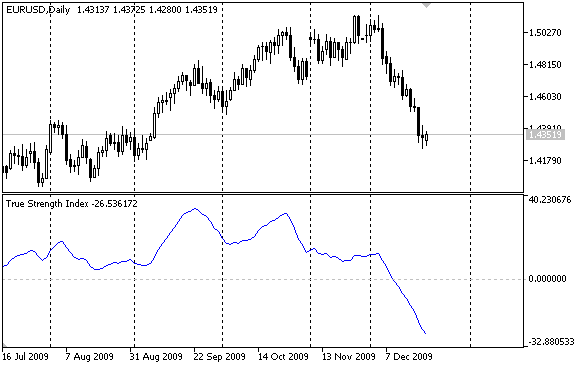Still there are some questions left.

### Optimizing Calculations

Actually, it's not enough just to write a working indicator. If we carefully look at the current implementation of OnCalculate(), we'll see that it's not optimal.

```int OnCalculate (const int rates_total,    // size of the price[] array;
const int prev_calculated,// number of available bars;
// at the previous call;
const int begin,// from what index of the
// price[] array true data start;
const double &price[]) // array, at which the indicator will be calculated;
{
//--- calculate values of mtm and |mtm|
MTMBuffer=0.0;
AbsMTMBuffer=0.0;
for(int i=1;i<rates_total;i++)
{
MTMBuffer[i]=price[i]-price[i-1];
AbsMTMBuffer[i]=fabs(MTMBuffer[i]);
}
//--- calculate the first moving average on arrays
ExponentialMAOnBuffer(rates_total,prev_calculated,
1,  // index, starting from which data for smoothing are available
r,  // period of the exponential average
MTMBuffer,       // buffer to calculate average
EMA_MTMBuffer);  // into this buffer locate value of the average
ExponentialMAOnBuffer(rates_total,prev_calculated,
1,r,AbsMTMBuffer,EMA_AbsMTMBuffer);

//--- calculate the second moving average on arrays
ExponentialMAOnBuffer(rates_total,prev_calculated,
r,s,EMA_MTMBuffer,EMA2_MTMBuffer);
ExponentialMAOnBuffer(rates_total,prev_calculated,
r,s,EMA_AbsMTMBuffer,EMA2_AbsMTMBuffer);
//--- now calculate values of the indicator
for(int i=r+s-1;i<rates_total;i++)
{
TSIBuffer[i]=100*EMA2_MTMBuffer[i]/EMA2_AbsMTMBuffer[i];
}
//--- return value of prev_calculated for next call
return(rates_total);
}```

At each function start we calculate values in arrays of MTMBuffer[] and AbsMTMBuffer[]. In this case, if the size of price[] equals to hundreds of thousands or even millions, unnecessary repeated calculations can take all CPU resources, no matter how powerful it is.

For organizing optimal calculations, we use the prev_calculated input parameter, which is equal to the value returned by OnCalculate() at the previous call. In the first call of the function, the value of prev_calculated is always equal to 0. In this case we calculate all values in the indicator buffer. During the next call, we won't have to calculate the whole buffer - only the last value will be calculated. Let's write it down like this:

```//--- if it is the first call
if(prev_calculated==0)
{
//--- set zero values to zero indexes
MTMBuffer=0.0;
AbsMTMBuffer=0.0;
}
//--- calculate values of mtm and |mtm|
int start;
if(prev_calculated==0) start=1;  // start filling out MTMBuffer[] and AbsMTMBuffer[] from the 1st index
else start=prev_calculated-1;    // set start equal to the last index in the arrays
for(int i=start;i<rates_total;i++)
{
MTMBuffer[i]=price[i]-price[i-1];
AbsMTMBuffer[i]=fabs(MTMBuffer[i]);
}```

Calculation blocks of EMA_MTMBuffer[], EMA_AbsMTMBuffer[], EMA2_MTMBuffer[] and EMA2_AbsMTMBuffer[] do not require optimization of calculations, because ExponentialMAOnBuffer() is already written in the optimal way. We need to optimize only calculation of values for the TSIBuffer[] array. We use the same method as the one, used for MTMBuffer[].

```//--- now calculate the indicator values
if(prev_calculated==0) start=r+s-1; // set the starting index for input arrays
for(int i=start;i<rates_total;i++)
{
TSIBuffer[i]=100*EMA2_MTMBuffer[i]/EMA2_AbsMTMBuffer[i];
}
//--- return value of prev_calculated for next call
return(rates_total);```

The last remark for the optimization procedure: OnCalculate() returns the value of rates_total. This means the number of elements in the price[] input array, which is used for indicator calculations.

Value returned by OnCalculate() is saved in the terminal memory, and at the next call of OnCalculate() it is passed to the function as the value of the input parameter prev_calculated.

This allows to always know the size of the input array at the previous call of OnCalculate() and start the calculation of indicator buffers from a correct index without unnecessary recalculations.

### Checking Input Data

There is one more thing we need to do for OnCalculate() to operate perfectly. Let's add checking of the price[] array, on which indicator values are calculated. If the size of the array (rates_total) is too small, no calculations are required - we need to wait till the next call of OnCalculate(), when data are enough.

```//--- if the size of price[] is too small
if(rates_total<r+s) return(0); // do not calculate or draw anything
//--- if it's the first call
if(prev_calculated==0)
{
//--- set zero values for zero indexes
MTMBuffer=0.0;
AbsMTMBuffer=0.0;
}```

Since the exponential smoothing is used twice sequentially to calculate True Strength Index, the size of price[] must be at least equal to or larger than the sum of r and s periods; otherwise the execution is terminated, and OnCalculate() returns 0. The returned zero value means that the indicator will not be plotted in the chart, because its values aren't calculated.

### Setting up Representation

As for the correctness of calculations, the indicator is ready to use. But if we call it from another mql5-program, it will be built by Close prices on default. We can specify another default price type - specify a value from the ENUM_APPLIED_PRICE enumeration in the indicator_applied_price property of the indicator.

For example, in order to set a typical price ( (high+low+close)/3) for a price, let's write the following:

#property indicator_applied_price PRICE_TYPICAL

If we are planning to use only its values using iCustom() or IndicatorCreate() functions, no further refinements are required. But if used directly, i.e. plotted in the chart, additional settings are recommended:

• bar number, starting from which an indicator is plotted;
• Label for values in TSIBuffer[], which will be reflected in DataWindow;
• short name of the indicator, shown in a separate window and in the pop-up help when pointing the mouse cursor over the indicator line;
• number of digits after the decimal point that is shown in the indicator values (this doesn't affect the accuracy).

These settings can be tuned in the OnInit() handler, using functions from the Custom Indicators group. Add new lines and save the indicator as True_Strength_Index_ver2.mq5.

```//+------------------------------------------------------------------+
//| Custom indicator initialization function                         |
//+------------------------------------------------------------------+
int OnInit()
{
//--- indicator buffers mapping
SetIndexBuffer(0,TSIBuffer,INDICATOR_DATA);
SetIndexBuffer(1,MTMBuffer,INDICATOR_CALCULATIONS);
SetIndexBuffer(2,AbsMTMBuffer,INDICATOR_CALCULATIONS);
SetIndexBuffer(3,EMA_MTMBuffer,INDICATOR_CALCULATIONS);
SetIndexBuffer(4,EMA2_MTMBuffer,INDICATOR_CALCULATIONS);
SetIndexBuffer(5,EMA_AbsMTMBuffer,INDICATOR_CALCULATIONS);
SetIndexBuffer(6,EMA2_AbsMTMBuffer,INDICATOR_CALCULATIONS);
//--- bar, starting from which the indicator is drawn
PlotIndexSetInteger(0,PLOT_DRAW_BEGIN,r+s-1);
string shortname;
StringConcatenate(shortname,"TSI(",r,",",s,")");
//--- set a label do display in DataWindow
PlotIndexSetString(0,PLOT_LABEL,shortname);
//--- set a name to show in a separate sub-window or a pop-up help
IndicatorSetString(INDICATOR_SHORTNAME,shortname);
//--- set accuracy of displaying the indicator values
IndicatorSetInteger(INDICATOR_DIGITS,2);
//---
return(0);
}```

If we start both versions of the indicator and scroll the chart to the beginning, we'll see all the differences.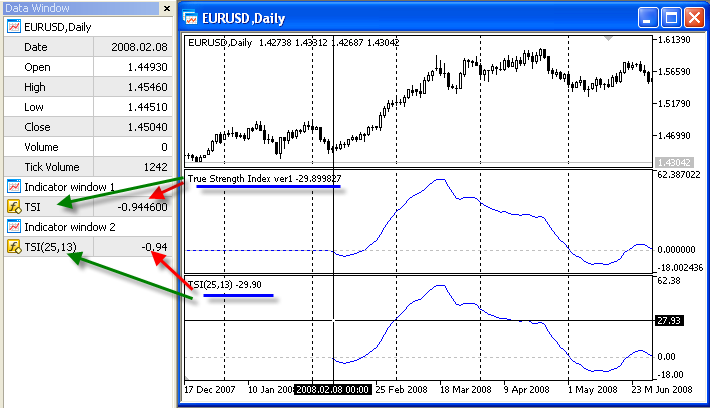### Conclusion

Based on the example of creating the True Strength Index indicator, we can outline the basic moments in the process of writing of any indicator in MQL5:

• To create your own custom indicator use MQL5 Wizard that will help you perform preliminary routine operations on the indicator setup. Select the necessary variant of the OnCalculate() function.
• If necessary, add more arrays for intermediate calculations and bind them with corresponding indicator buffers using the SetIndexBuffer() function. Indicate the INDICATOR_CALCULATIONS type for these buffers.
• Optimize calculations in OnCalculate(), because this function will be called each time price data change. Use ready debugged functions to make code writing easier, and for better readability.
• Perform additional visual tuning of the indicator, to make the program easy to use both for other mql5 programs and by users.

Translated from Russian by MetaQuotes Ltd.
Original article: https://www.mql5.com/ru/articles/10

Attached files |

#### Other articles by this author

Last comments | Go to discussion (6)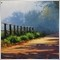| 12 May 2010 at 18:15

Very good! Thank you !

Teach our newbies step by step.

I have a question,

is it necessary or more proper to add the below code ?

ArraySetAsSeries(price,true);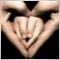| 22 Aug 2010 at 07:43

for(int i=1;i<rates_total;i++)
{
MTMBuffer[i]=price[i]-price[i-1];
AbsMTMBuffer[i]=fabs(MTMBuffer[i]);
}

Why use [i-1] to calculate [i] and start i=1 ?  no  ?

MTMBuffer[i]=price[i]-price[i-1];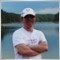| 22 Aug 2010 at 10:06| 9 Dec 2021 at 04:06

I am with @okwh. I am still baffled why the loop starts with 1?

I've read the article that was pointed by @Rashid Umarov. It did say this :

The default indexing of all arrays and indicator buffers is left to right. The index of the first element is always equal to zero. Thus, the very first element of an array or indicator buffer with index 0 is by default on the extreme left position, while the last element is on the extreme right position.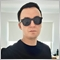| 14 Jul 2022 at 23:25
Thank your very much for this useful article. As a beginner using the MT5 platform, and as @Wolfgang pointed out, those of us who are not professional coders really depend on these types of articles to get familiarized with the inner workings of the language.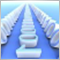Data Exchange between Indicators: It's Easy
We want to create such an environment, which would provide access to data of indicators attached to a chart, and would have the following properties: absence of data copying; minimal modification of the code of available methods, if we need to use them; MQL code is preferable (of course, we have to use DLL, but we will use just a dozen of strings of C++ code). The article describes an easy method to develop a program environment for the MetaTrader terminal, that would provide means for accessing indicator buffers from other MQL programs.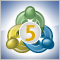Here Comes the New MetaTrader 5 and MQL5
This is just a brief review of MetaTrader 5. I can't describe all the system's new features for such a short time period - the testing started on 2009.09.09. This is a symbolical date, and I am sure it will be a lucky number. A few days have passed since I got the beta version of the MetaTrader 5 terminal and MQL5. I haven't managed to try all its features, but I am already impressed.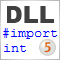How to Exchange Data: A DLL for MQL5 in 10 Minutes
Now not so many developers remember how to write a simple DLL, and what are special features of different system binding. Using several examples, I will try to show the entire process of the simple DLL's creation in 10 minutes, as well as to discuss some technical details of our binding implementation. I will show the step-by-step process of DLL creation in Visual Studio with examples of exchanging different types of variables (numbers, arrays, strings, etc.). Besides I will explain how to protect your client terminal from crashes in custom DLLs.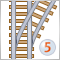Step on New Rails: Custom Indicators in MQL5
I will not list all of the new possibilities and features of the new terminal and language. They are numerous, and some novelties are worth the discussion in a separate article. Also there is no code here, written with object-oriented programming, it is a too serous topic to be simply mentioned in a context as additional advantages for developers. In this article we will consider the indicators, their structure, drawing, types and their programming details, as compared to MQL4. I hope that this article will be useful both for beginners and experienced developers, maybe some of them will find something new.